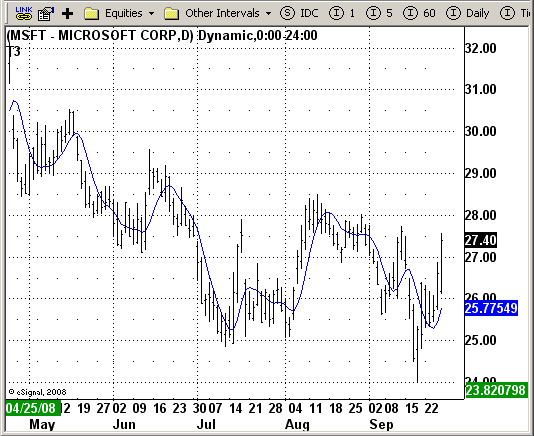# T3 Average

ICE Data Services -

 T3Avg.efs EFSLibrary - Discussion Board

File Name: T3Avg.efs

Description:
T3 Average

Formula Parameters:
Length: 5
Price Data To Use: Close

Notes:
This indicator plots the moving average described in the January, 1998 issue
of S&C, p.57, "Smoothing Techniques for More Accurate Signals", by Tim Tillson.
This indicator plots T3 moving average presented in Figure 4 in the article.
T3 indicator is a moving average which is calculated according to formula:
T3(n) = GD(GD(GD(n))),
where GD - generalized DEMA (Double EMA) and calculating according to this:
GD(n,v) = EMA(n) * (1+v)-EMA(EMA(n)) * v,
where "v" is volume factor, which determines how hot the moving averageRs response
to linear trends will be. The author advises to use v=0.7.
When v = 0, GD = EMA, and when v = 1, GD = DEMA. In between, GD is a less aggressive
version of DEMA. By using a value for v less than1, trader cure the multiple DEMA
overshoot problem but at the cost of accepting some additional phase delay.
In filter theory terminology, T3 is a six-pole nonlinear Kalman filter. Kalman
filters are ones that use the error W in this case, (time series - EMA(n)) W
to correct themselves. In the realm of technical analysis, these are called adaptive
moving averages; they track the time series more aggres-sively when it is making large
moves. Tim Tillson is a software project manager at Hewlett-Packard, with degrees in
mathematics and computer science. He has privately traded options and equities for 15 years.`/*********************************Provided By: eSignal (Copyright c eSignal), a division of Interactive Data Corporation. 2008. All rights reserved. This sample eSignal Formula Script (EFS) is for educational purposes only and may be modified and saved under a new file name. eSignal is not responsible for the functionality once modified. eSignal reserves the right to modify and overwrite this EFS file with each new release.Description: T3 Average Version: 1.0 09/24/2008Notes: This indicator plots the moving average described in the January, 1998 issue of S&C, p.57, "Smoothing Techniques for More Accurate Signals", by Tim Tillson. This indicator plots T3 moving average presented in Figure 4 in the article. T3 indicator is a moving average which is calculated according to formula: T3(n) = GD(GD(GD(n))), where GD - generalized DEMA (Double EMA) and calculating according to this: GD(n,v) = EMA(n) * (1+v)-EMA(EMA(n)) * v, where "v" is volume factor, which determines how hot the moving averageRs response to linear trends will be. The author advises to use v=0.7. When v = 0, GD = EMA, and when v = 1, GD = DEMA. In between, GD is a less aggressive version of DEMA. By using a value for v less than1, trader cure the multiple DEMA overshoot problem but at the cost of accepting some additional phase delay. In filter theory terminology, T3 is a six-pole nonlinear Kalman filter. Kalman filters are ones that use the error W in this case, (time series - EMA(n)) W to correct themselves. In the realm of technical analysis, these are called adaptive moving averages; they track the time series more aggres-sively when it is making large moves. Tim Tillson is a software project manager at Hewlett-Packard, with degrees in mathematics and computer science. He has privately traded options and equities for 15 years. Formula Parameters: Default: Length 5 Price Data To Use Close**********************************/var fpArray = new Array();var bInit = false;function preMain() { setPriceStudy(true); setStudyTitle("T3"); setCursorLabelName("Slope", 0); setDefaultBarFgColor(Color.blue, 0); var x=0; fpArray[x] = new FunctionParameter("Length", FunctionParameter.NUMBER); with(fpArray[x++]){ setLowerLimit(1); setDefault(5); } fpArray[x] = new FunctionParameter("Price", FunctionParameter.STRING); with(fpArray[x++]){ setName("Price Data To Use"); addOption("open"); addOption("high"); addOption("low"); addOption("close"); addOption("hl2"); addOption("hlc3"); addOption("ohlc4"); setDefault("close"); }}var xe1 = null;var xe2 = null;var xe3 = null;var xe4 = null;var xe5 = null;var xe6 = null;var xMyPrice = null;var nT3Average = 0;function main(Price, Length) {var nState = getBarState(); if (nState == BARSTATE_ALLBARS) { if (Price == null) Price = "close"; if (Length == null) Length = 5; } if ( bInit == false ) { xMyPrice = eval(Price)(); xe1 = ema(Length, xMyPrice); xe2 = ema(Length, xe1); xe3 = ema(Length, xe2); xe4 = ema(Length, xe3); xe5 = ema(Length, xe4); xe6 = ema(Length, xe5); bInit = true; } if (xe1.getValue(0) == null || xe2.getValue(0) == null || xe3.getValue(0) == null || xe4.getValue(0) == null || xe5.getValue(0) == null || xe6.getValue(0) == null) return; var b = 0.7; var c1 = -b*b*b; var c2 = 3*b*b+3*b*b*b; var c3 = -6*b*b-3*b-3*b*b*b; var c4 = 1+3*b+b*b*b+3*b*b; nT3Average = c1 * xe6.getValue(0) + c2 * xe5.getValue(0) + c3 * xe4.getValue(0) + c4 * xe3.getValue(0); return nT3Average;}`